# Garden Hose Flow Rate and Time

The amount of water flow from a garden hose. This assumes a free-flowing hose end (not connected to a sprinkler or something). The minutes to supply a given volume is also determined below. Learn more about the units used on this page.

## Water Flow Rate

Hose Size:
in
Hose Supply Pressure:
psi
Hose Length:
feet
Water Flow Rate:

Volume:
Time:

## The Equations

This flow calculator uses the Hazen Williams equation to solve for flow assuming that the pressure at the outlet is zero (open to atmosphere) and thus the entire pressure at the hose bib is dissipated by friction losses in the hose. The pressure at the hose bib is assumed to be the dynamic pressure (while water is flowing) not the static pressure (no water flowing). Note that at high flow rates the dynamic pressure at the hose bib often drops because of internal pressure losses in the water delivery system. The assumed C factor (internal to the equation constant) is 135 for flexible garden hoses.

#### Water Flow RateWhere: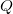= Water flow rate from the end of the hose (gpm)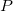= Pressure at the hose bib while hose end is free flowing (dynamic pressure, not static pressure) (psi)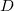= Hose internal diameter (in)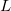= The hose length (ft)

#### Water Flow Time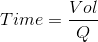Where:= Time it will take to fill the specified volume (min)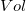= Volume needed (gal)= Flow rate from the garden hose (gpm)

WSU Prosser - IAREC, 24106 N Bunn Rd, Prosser WA 99350-8694, 509-786-2226. Contact Us